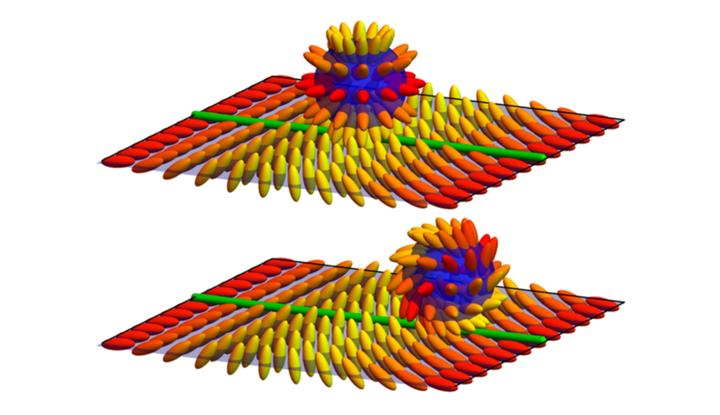# Homotopy characterization of non-Hermitian Hamiltonians### Abstract

We revisit the problem of classifying topological band structures in non-Hermitian systems. Recently, a solution has been proposed, which is based on redefining the notion of energy band gap in two different ways, leading to the so-called “point-gap” and “line-gap” schemes. However, simple Hamiltonians without band degeneracies can be constructed which correspond to neither of the two schemes. Here, we resolve this shortcoming of the existing classifications by developing the most general topological characterization of non-Hermitian bands for systems without a symmetry. Our approach, which is based on homotopy theory, makes no particular assumptions on the band gap, and predicts significant extensions to the previous classification frameworks. In particular, we show that the one-dimensional invariant generalizes from $\mathbb{Z}$ winding number to the non-Abelian braid group, and that depending on the braid group invariants, the two-dimensional invariants can be cyclic groups $\mathbb{Z}_n$ (rather than $\mathbb{Z}$ Chern number). We interpret these results in terms of a correspondence with gapless systems, and we illustrate them in terms of analogies with other problems in band topology, namely, the fragile topological invariants in Hermitian systems and the topological defects and textures of nematic liquids.

Publication
Phys. Rev. B 101, 205417 (2020)